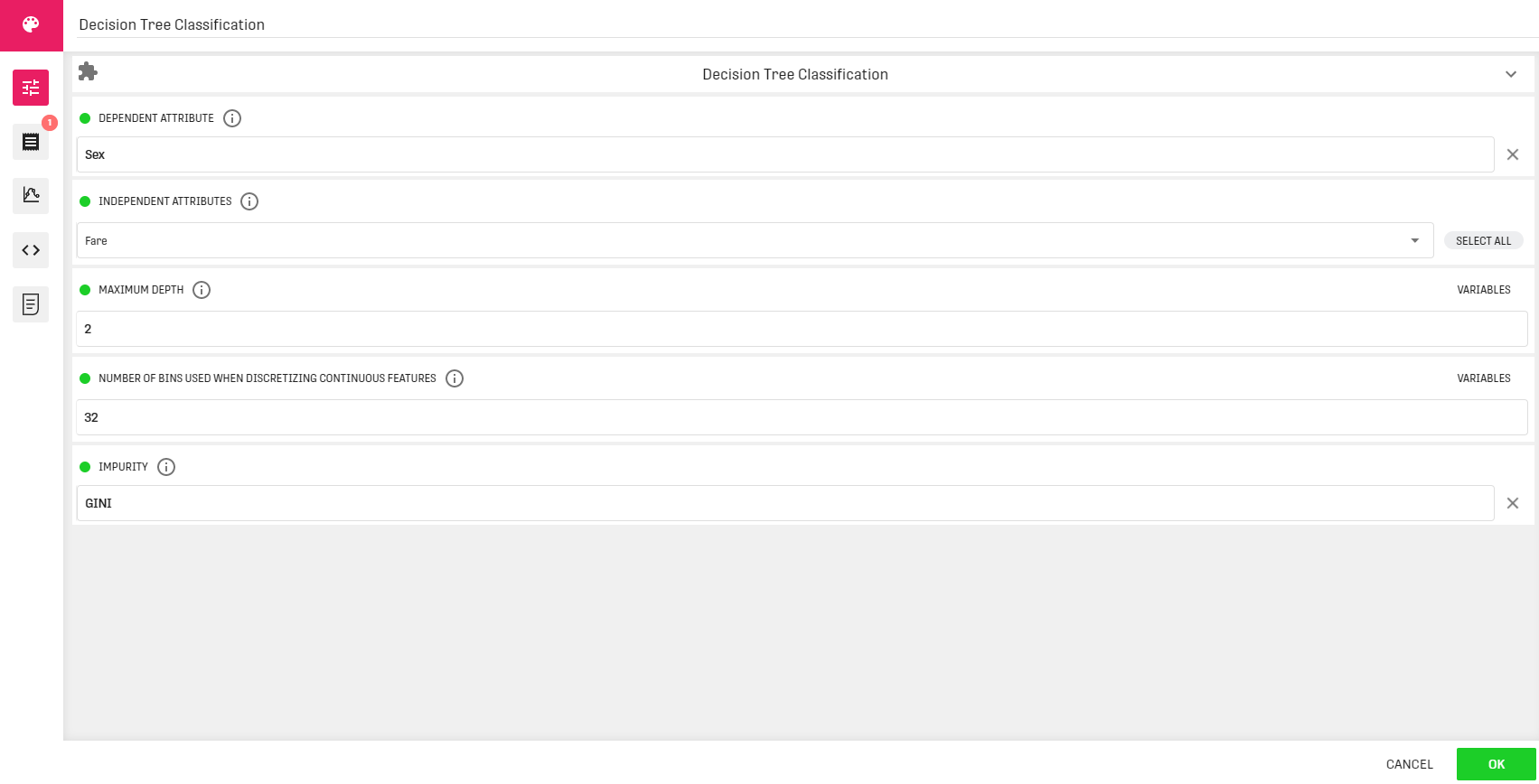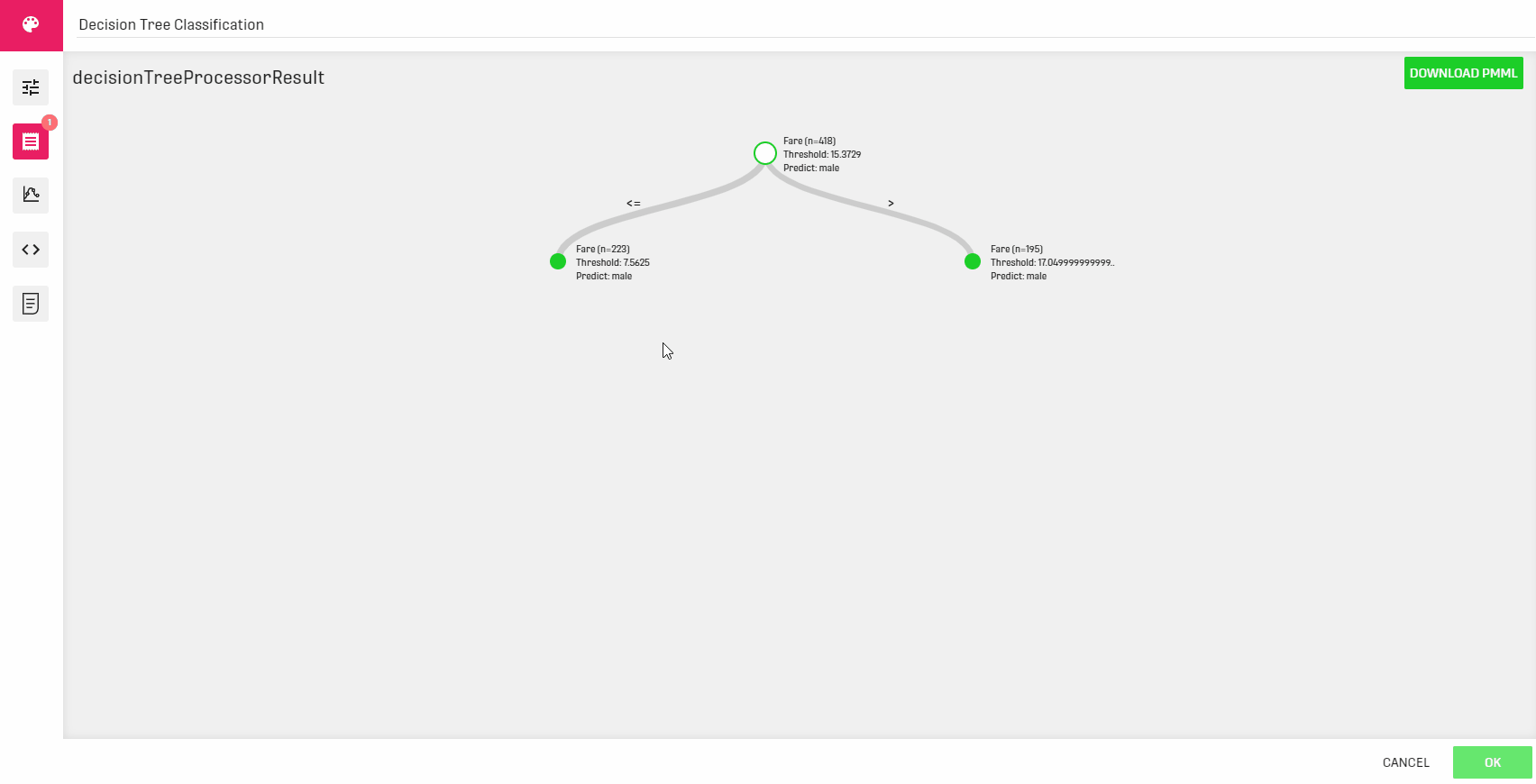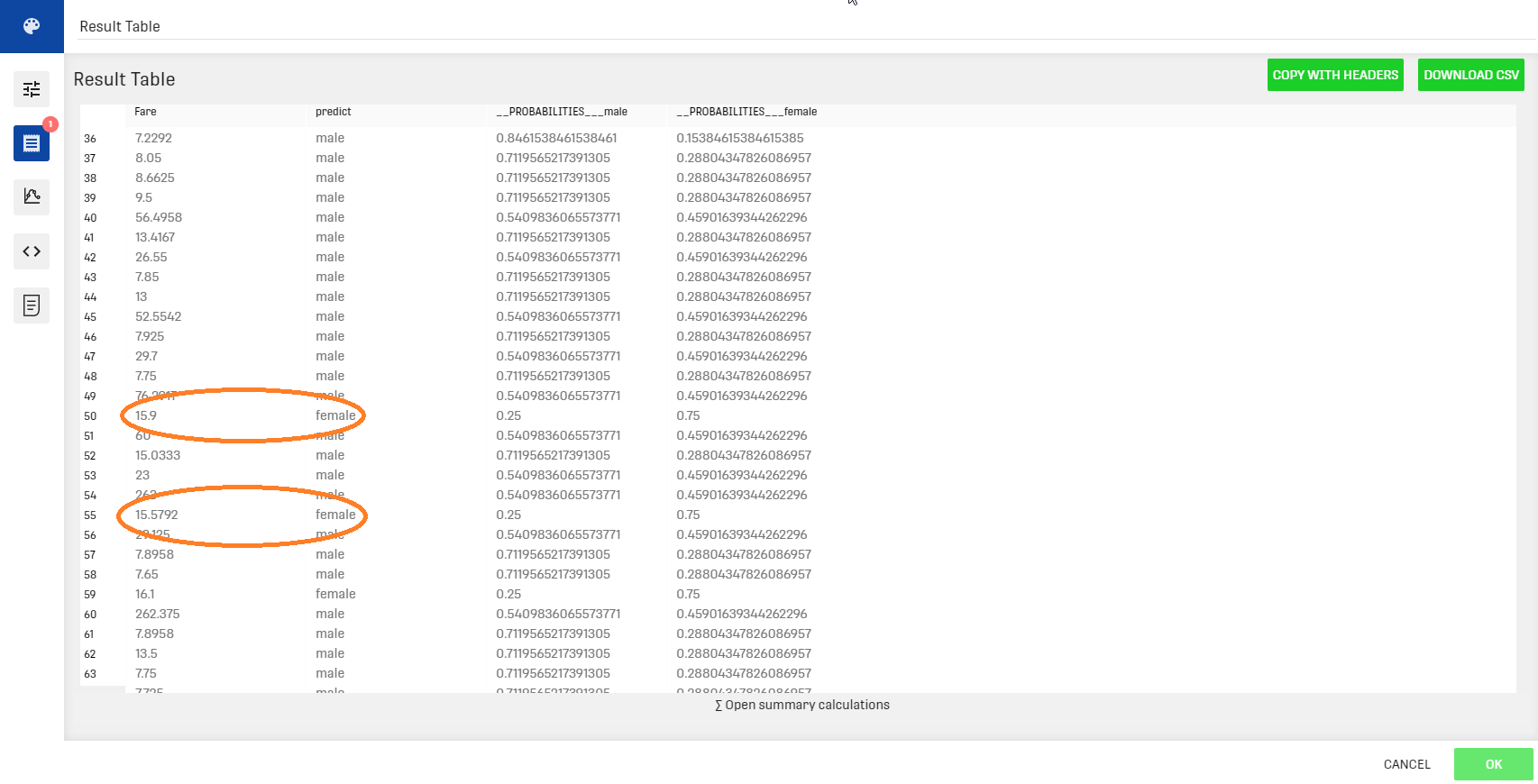# Decision Tree Classification Processor

Modified on Tue, 30 Nov 2021 at 03:46 PM

# Overview

This processor is used for creating a decision tree out of the given dataset.

`Note: Changed machine learning algorithm implementations in Spark 3 may slightly change results compared to Spark 2.`

## Different classification methods

The goal behind decision trees is always to find the best split for each node of the tree. But measuring the "goodness" of a given split is a subjective question so, in practice, different metrics are used for evaluating splits. One commonly used metric is the Entropy. Another common metric is the Gini impurity.

Further Information about decision trees can be found in the following link.

# Input

The processor requires an input that contains at least one categorical column which will be the subject of our classification.

# Output

The Decision Tree Classification Processor can provide two different outputs:

• A decision tree: this tree can be accessed through the decision tree classification processor under the "Results". It can also be downloaded in XML format.
• A probability table: a table presenting the predicted value along with the different probabilities. It can be viewed via the result table linked to the processor's output.

# Example

In this example, the input dataset represents information about train passengers (Name, Class, Age...). The goal is to build a decision tree that predicts the passenger's sex using the fare column.

## Example ConfigurationThe maximum depth here is set to 2 to provide a better visualization of the result tree. Needless to say, a higher depth value gives a better prediction (more splits).

Note that we are using the GINI Impurity in this example. Using the Entropy impurity will have the same configuration.

## Result

Decision treeResult tableNote that in the result table, the fare values 15.9 and 15.58 are predicted to belong to female passengers. The same result can be interpreted by the given tree, as the third leaf (values between 15.3729 and 17.04999999..) is assigned the female prediction.

# Related Articles

Decision Tree Classification Forecast Processor

Decision Tree Regression Processor

Decision Tree Regression Forecast Processor